ILNumerics - Technical Computing

Modern High Performance Tools for Technical

Computing and Visualization in Industry and Science

# Live Debug Data Plotting in C#

ILNumerics Array Visualizer is an interactive, graphical debug tool for Visual Studio. During your debug session it connects to the Visual Studio debug engine and allows immediate insight into the data of your C# program. Arbitrary array data is plotted in various, interactive chart styles: as 1D and 2D line plots, scatter plots, bar plots, as 3D surface plots, and images. Easily describe the data via arbitrary expressions. Export data in a large number of external formats, including charts, HDF5 and Microsoft Excel. Follow your data with each debug step to quickly find bugs in the algorithm.

## Quick Start

1. During your Visual Studio debug session go to VIEW -> Other Windows -> Array Visualizer.

2. Enter your expression into the textbox of the Array Visualizer Window.

3. Select the output type from the list of available 2D and 3D plots.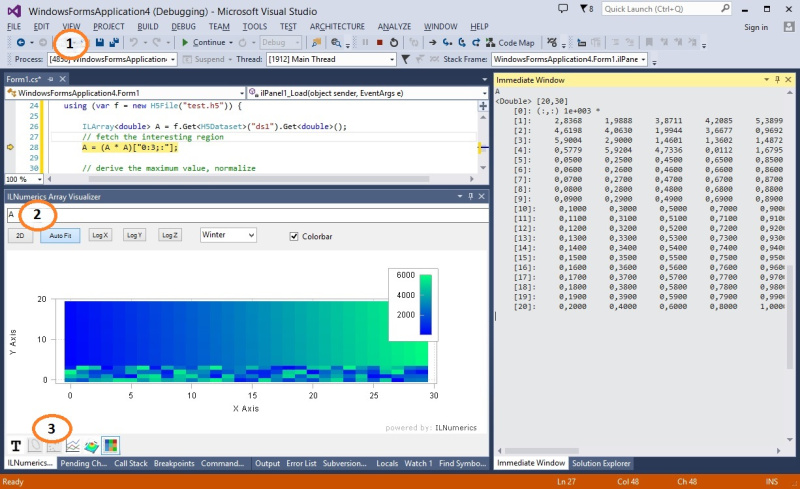## Plotting Array Expressions in C#

Let's start by creating a very simple C# program:

The content of the variable A at the current statement is plotted by entering A into the Array Visualizer and pressing [Enter].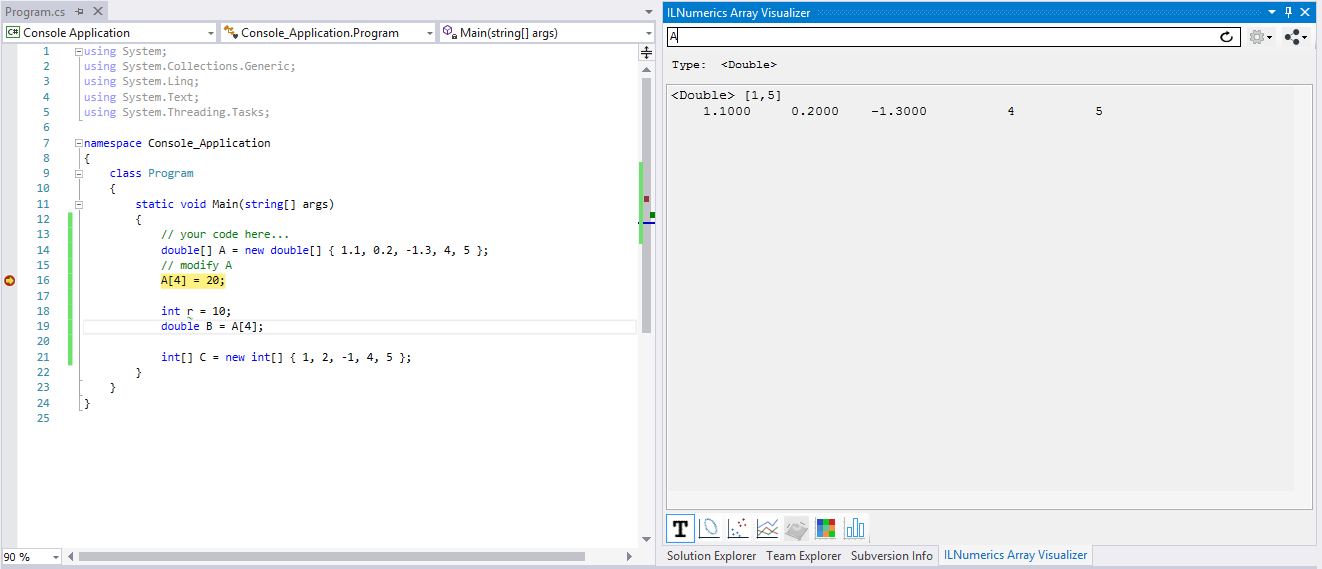Here, the content is shown as text. Click on the line plot tab on the bottom of the Array Visualizer tool window to show the data as line plot. Note how the plot is linked to the variable: pressing F10 modifies A in the next statement. The change is immediately reflected by the plot.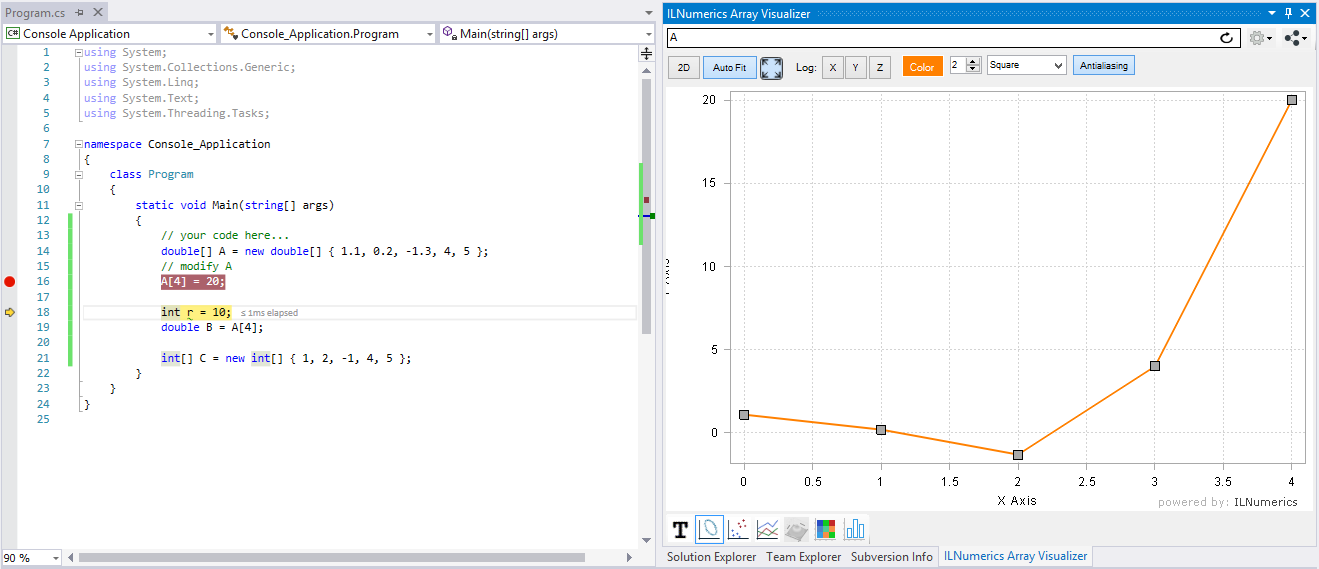### Getting Interactive

No matter, how you interact with the data in your debug session – changes will show up immediately in the array visualizer. One might set the 3rd element of A to the value 10 in the immediate window. See how the plot reflects the changes: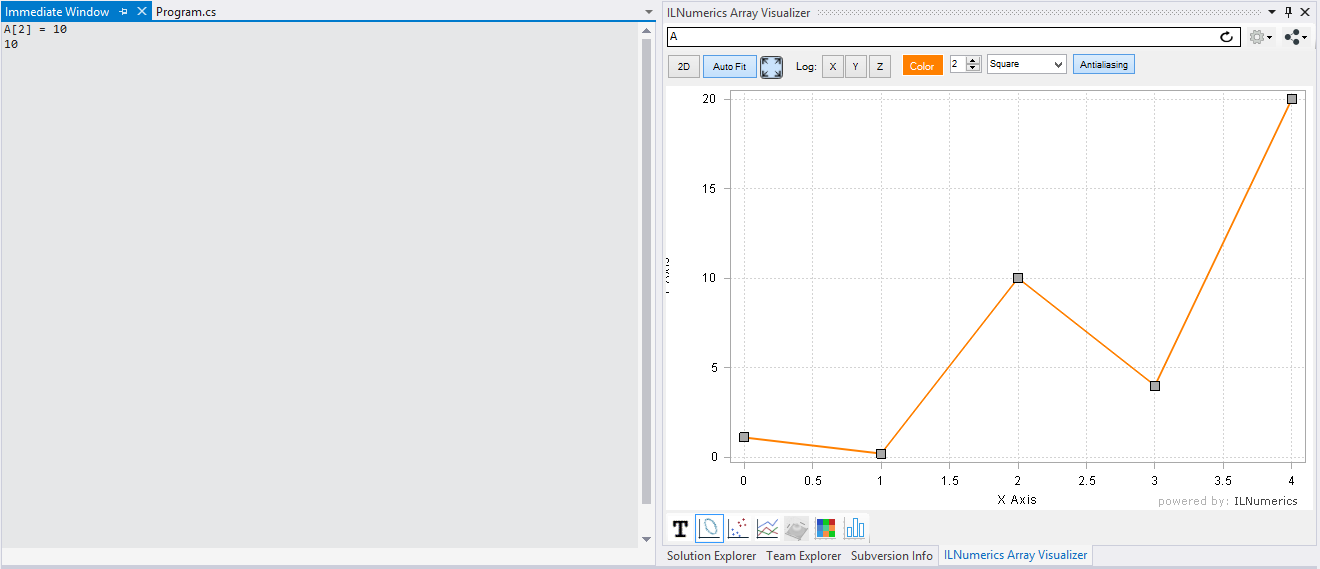Ad-hoc visualizations of any vector data are possible by entering code creating an array containing the desired elements. In order to plot some values, taken from constants, variables, other arrays or functions:

new double[]{ 1.2, A+1, -Math.Tan(-2.223), r, B }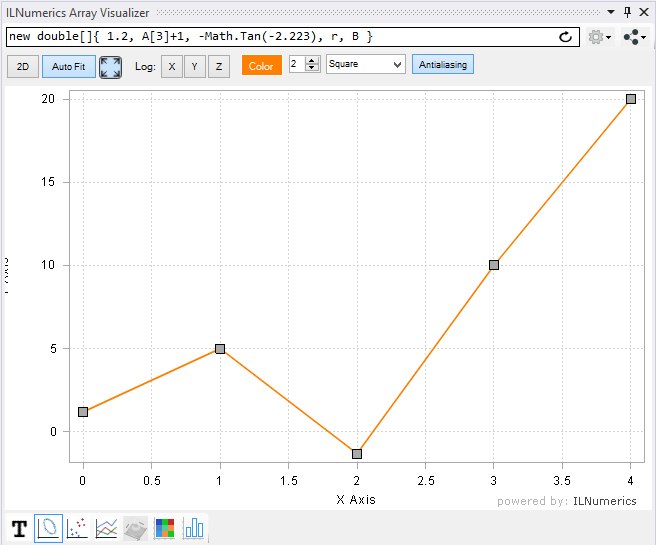### Supported Array Expressions in C#

In C# the ILNumerics Array Visualizer plots arbitrary expressions yielding the following array types:

• ILNumerics arrays: Array<T>, InArray<T>, ...
• 1D System.Array: double[], float[], int[], ILNumerics.complex(), ...
• Multidimensional System.Array: double[,,], int[,], byte[,,,], ILNumerics.complex(,), ...
• On-the-fly expressions evaluating to arrays: new int[] {1,2,3,4,5}fft(A)[r(0,end/2-1)], ...
• Arrays of structs ('records', user defined types, new in 4.11!)

All primitve numeric element types are supported:

 Floating point data types double float ILNumerics .complex ILNumerics .fcomplex Integral data types int long short sbyte uint ulong ushort byte Character char

Expression entered in the Array Visualizer are evaluated in the context of the current debug stack frame. Your program must be halted in debug mode. Any valid C# code can be used as expression for the Array Visualizer, including variables, function calls etc.

Users of the ILNumerics Computing Engine can utilize any function from ILMath, from any referenced toolbox, combining several Array<T> variables, subarray expressions, accessing HDF5 data, and so on...

### N-D Array Plotting Example

Handling matrices and arrays with more dimensions works in the same way.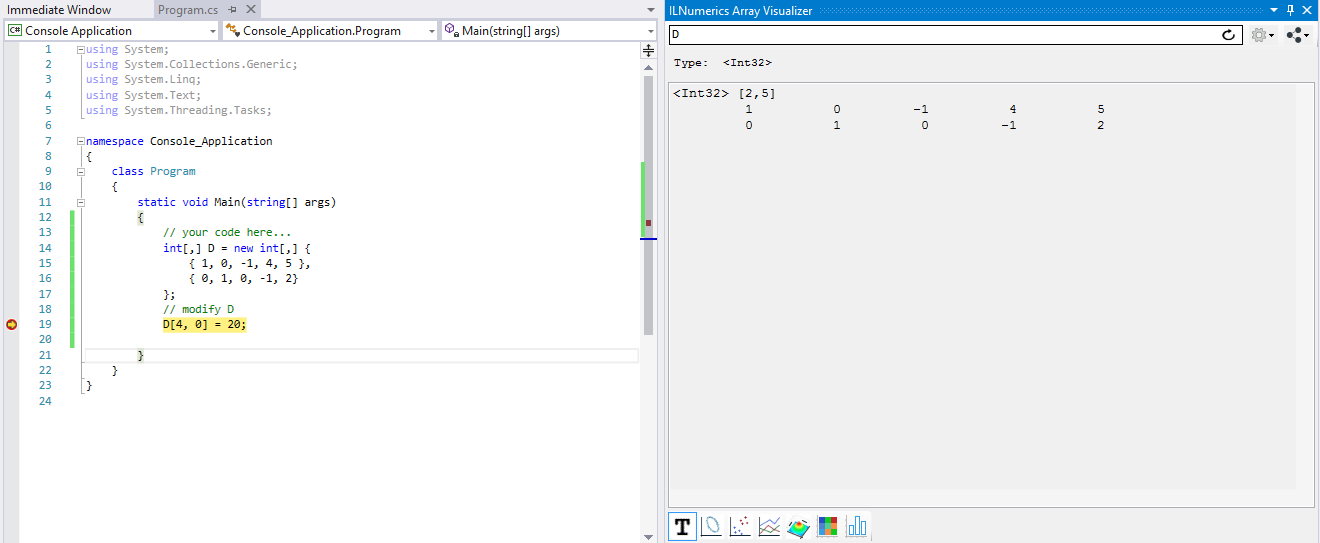## Plot Types

Next to the text output, the 2D matrix D can be visualized by help of the following chart types:

 XY-Line Plot Scatter Plot Rows Plot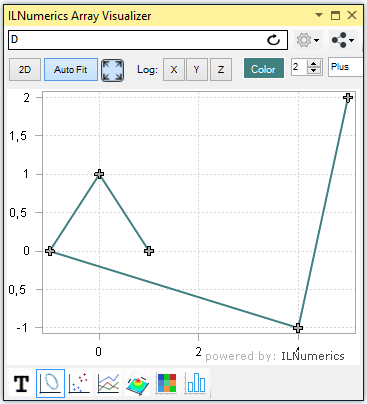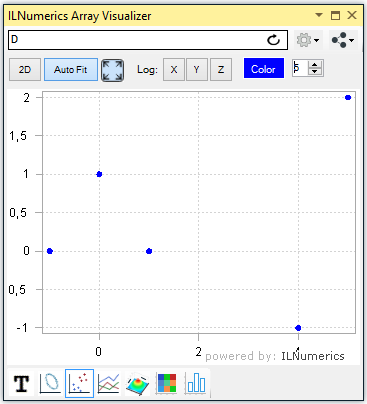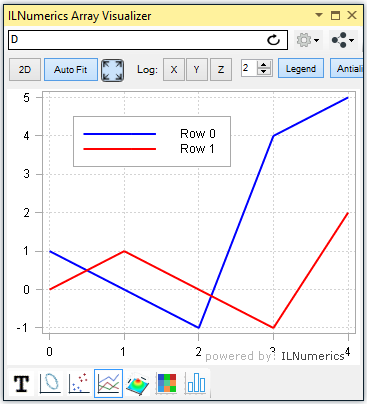Surface Plot ImageSC Plot Bar Plot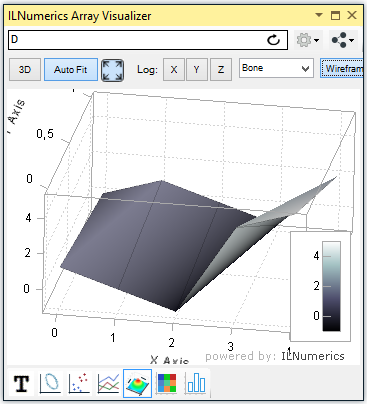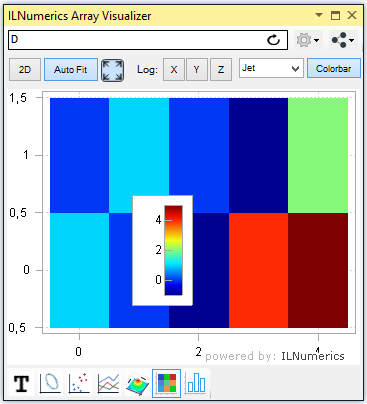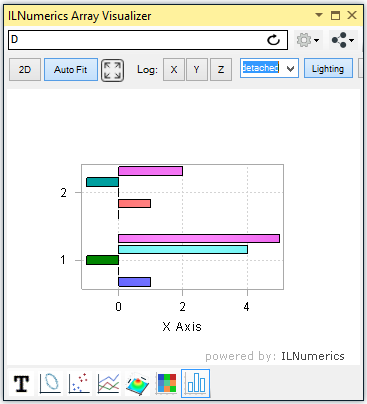The row of iconized buttons on the bottom side of the the Array Visualizer tool window represents individual plot types. Each plot provides a different view of the data. Read more

## Advanced C# Data Visualization

### Using Array Descriptors in C#

In the previous sections of this manual, the data to plot were represented as full dense array variables. However, many technical applications implement more complex data storage scenarios. In most situations, the interesting data are stored within recordsets, or structs of more complex data types.

Example: potential data type definition for storing 3D measurement data.

Here, 5 data elements of type double are stored for each measurement: the X,Y, and Z coordinates as the position of the measurement and two arbitrary data values. Data1 and Data2 might be used to store the actual value measured or some error range / confidence information computed for the measurement or any other useful value.

In real world applications data structures like this are very popular. Working with them can be cumbersome, however, especially if the number of measurements grows high. Developers often enough end up inspecting individual fields of every single measurement, utilizing data tips in Visual Studio: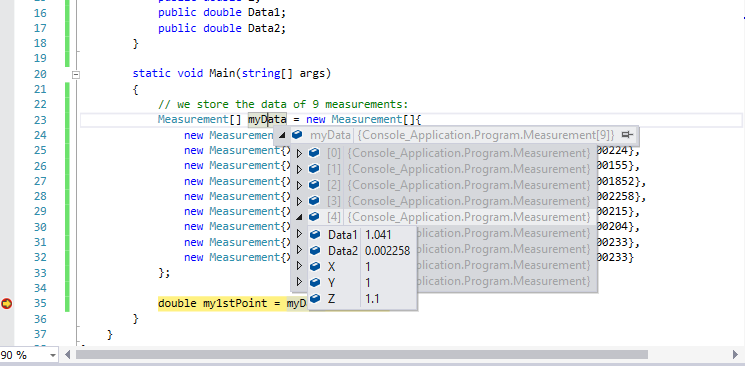Now, the ILNumerics Array Visualizer simplifies things significantly: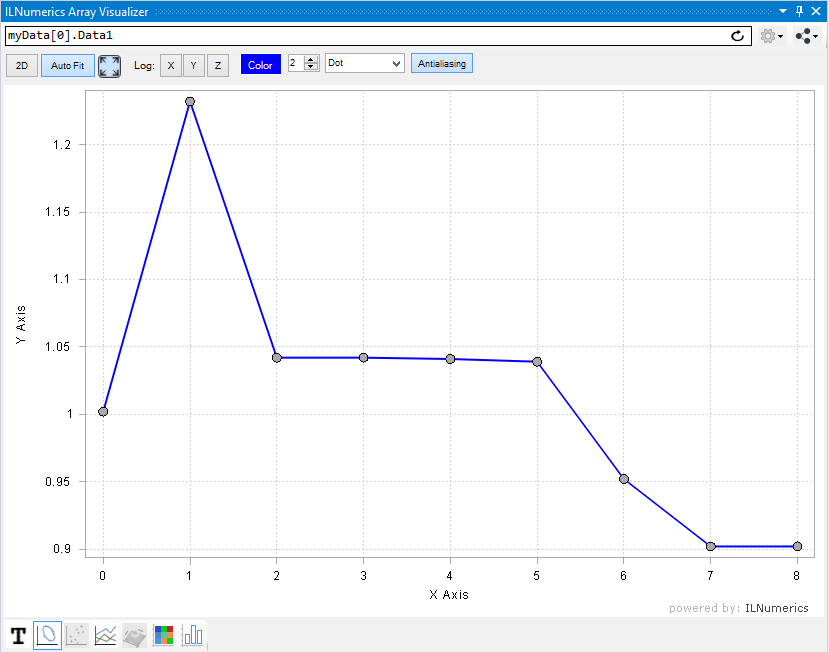Just enter the path to the interesting field, here: 'Data1'  into the expression text box and hit Enter. Choose a useful plotting type and inspect all Data1 values from all Measurements at once! In this example we selected 'Line Plot' as output type. Just as well, we could have made use of bar plots or others...

### Combining multiple members

With a little help of our flexible array descriptor syntax we can combine arbitrary elements of the structure array to create even more useful plots. Let's modify the expression to reference the array itself and press Enter: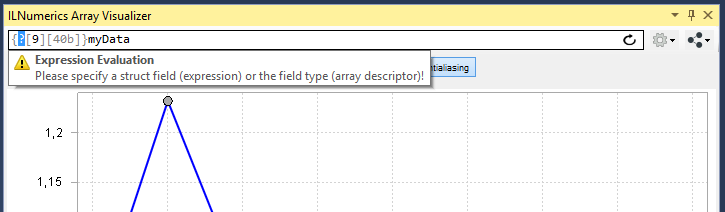The Array Visualizer recognizes that we wish to plot data from the struct array myData. It activated the array descriptor mode and filled in the length of the array (9) and the strides of individual elements for us (40b). Now, since struct arrays can have arbitrary member types, we are asked to enter a valid type for visualizing the data. Enter double as element type: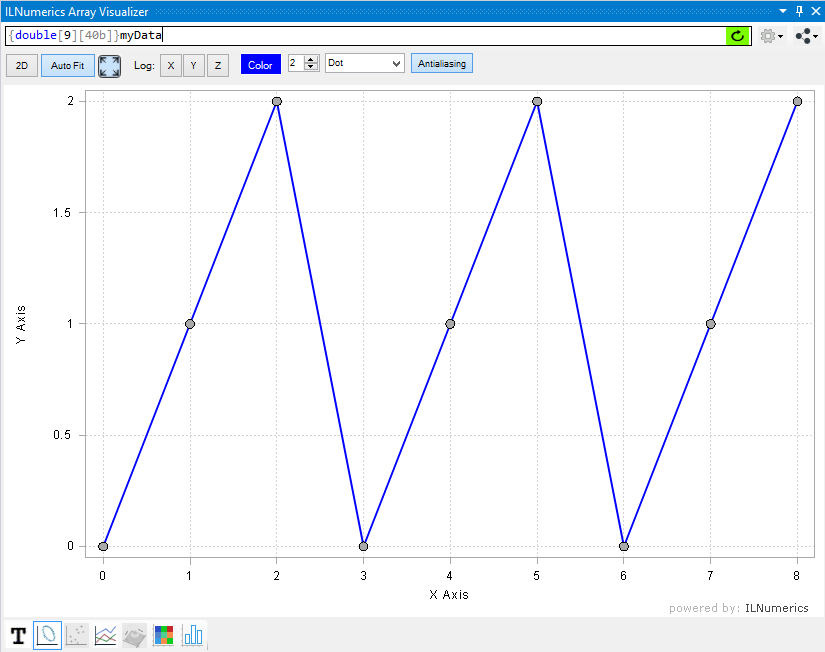What we see now is the visualization of the first struct member X of all array elements. We can enhance the plot by adding Y and Z. In order to archieve this, recall the memory layout of the struct array: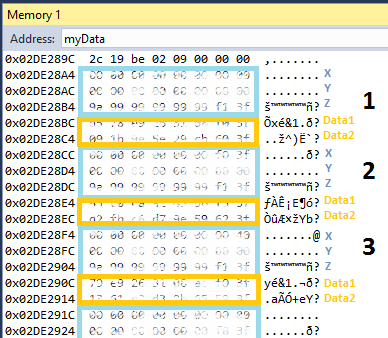By now we have extracted the vector of X values from the memory by picking the first double from each struct object, skipping over 40 bytes to get to the next X value. We may see this extraction as creating the following matrix row:

Extracting more data than X corresponds to having more rows in the matrix:

This requires a slight modification to the array descriptor only:

###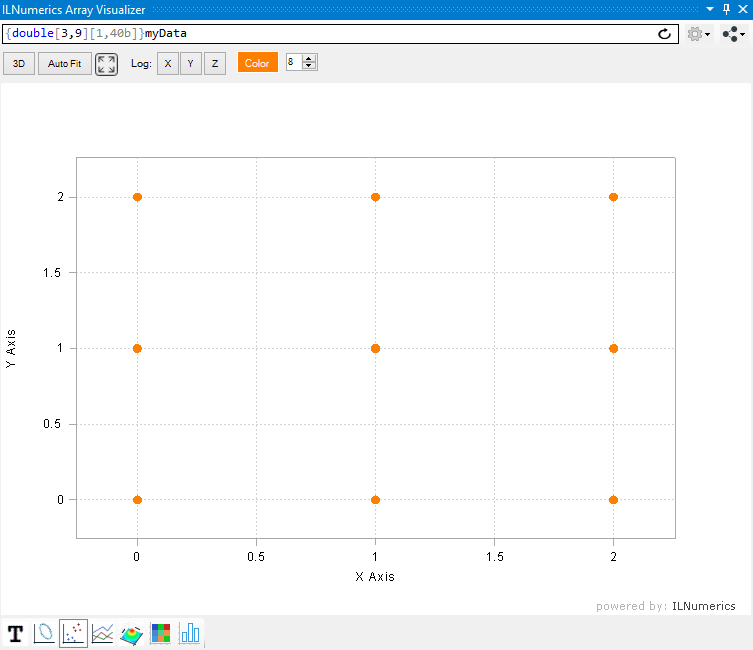This time and by using the scattered data plot the 3D positions of the measurements are made clearly visible. (We show the X-Y positions only here, since we look straight onto the X-Y plane. If there were any useful Z values we would visualize them by switching to the 3D view and rotating the plot with the mouse.)

Another useful view is given by the bar plot tab. It shows the X, Y and Z coordinates as separated groups: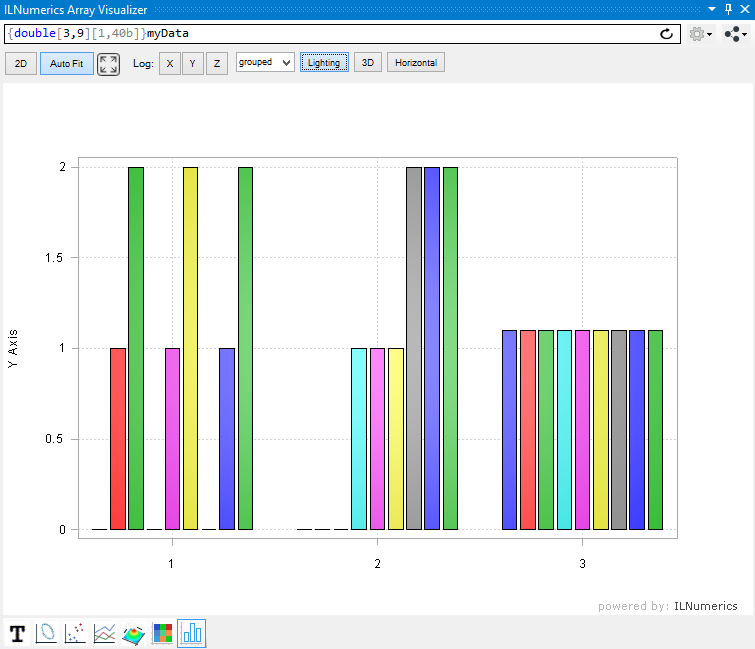Now we could easily add both, Data1 and Data2 as new rows to the matrix - this is left to the reader as exercise.

### Member Selection - Working with Offsets

Any member from the struct can be selected for plotting in the Array Visualizer individually. In order to plot both data members only we must jump over preceding elements and start the extraction matrix at the first (in terms of memory address) data member. In our example this corresponds to the starting address of the Data1 member of the 1st struct array element, 0x02DE28BC. This address is 3 x 8 = 24 bytes higher than the start of X1. We can use the array descriptor to enter the offset manually. Note that we also adjusted the lenght of the first dimension (2) to extract Data1 and the subsequent Data2 member: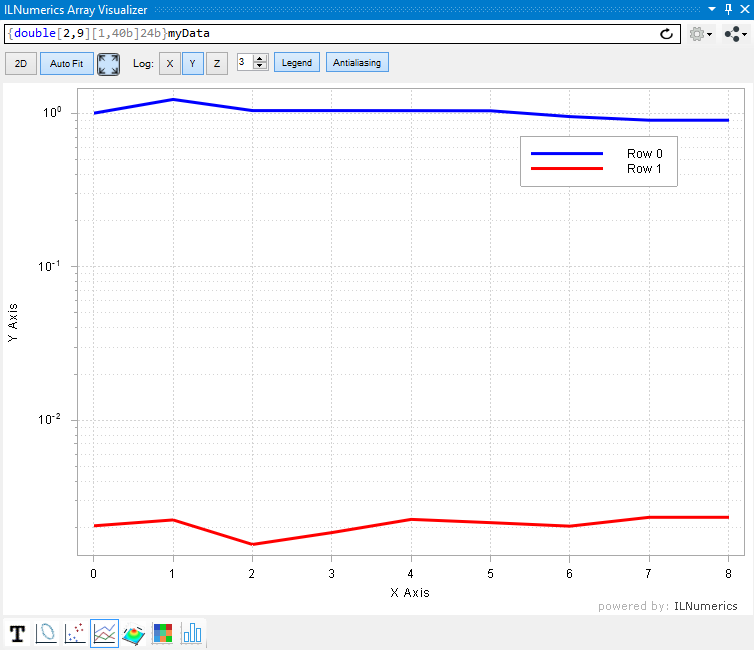Alternatively, we could leave the work of computing the required offset to the Array Visualizer. We start all over by clearing the Array Visualizer expression window (CTRL-A) and entering the direct path to the first Data1 member: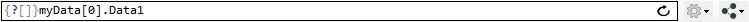->[Enter]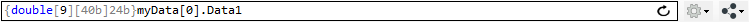Note how the offset of 24 bytes has been figured out automatically. From here, it is easy to add the additional dimension to get the exact same plotting result as in the example above: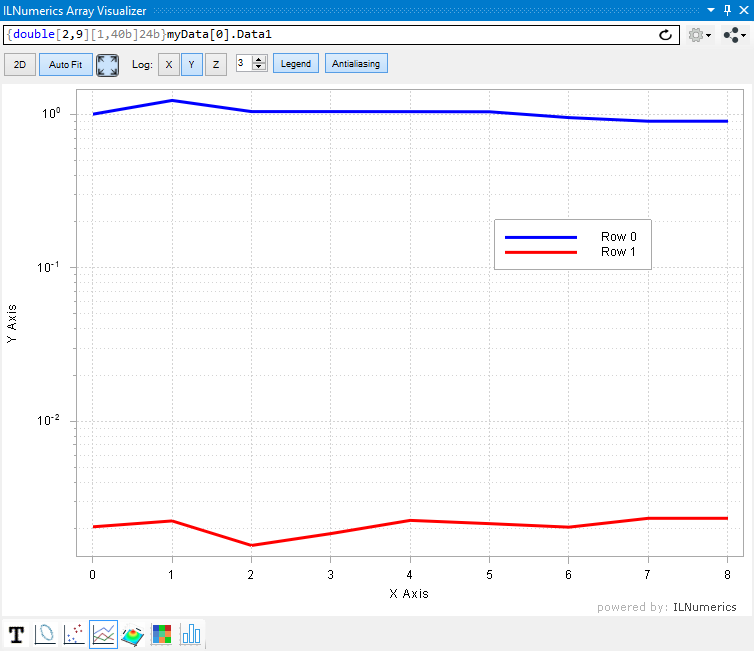### Replacing the Memory Window in C#

You may have encountered that the Visual Studio Debug Memory Window does not work as expected in all Situations in C#. Older versions of Visual Studio did not support memory display in C# at all. While more recent versions have improved, they still expose some problems in this matter. The Array Visualizer is able to display any accessible memory in any shape / type one may possibly needs. Therefore, it can be used as a nice replacement for the Debug Memory Tool Window.

Just select sbyte or byte as element type and provide the dimensions and strides as needed. The text view gives a nicely formatted overview table of the addressed memory region. Here, we visualize the bytes of a 3 x 4 x 5 Int32[,,] array in a table of 30 x 8 bytes: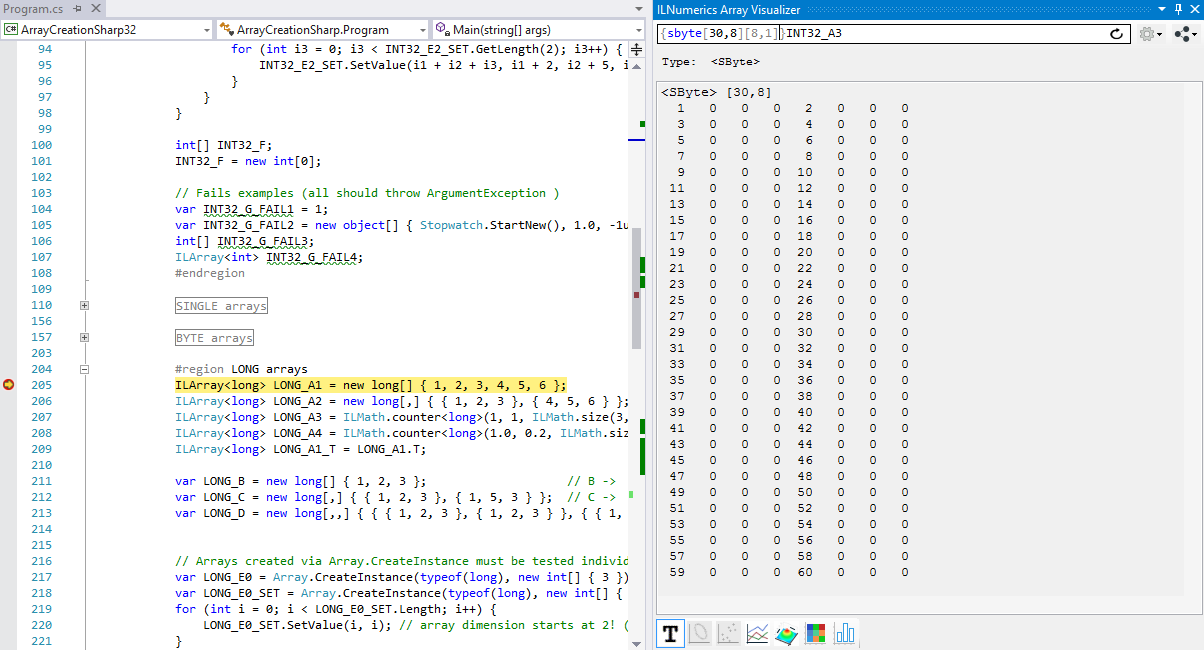Read more about array descriptors in the array descriptor tutorial (mixed languages).